Math & Beyond

# Paper Folding and Paper Cutting

22nd Sep '2021 views4 mins read

 1 Introduction 2 Example Problems 3 Practice Problems 4 Conclusion 5 Frequently Asked Questions (FAQs)

22 September 2020

Paper Folding and Cutting is a process in which a transparent sheet is folded and then a few cuts or folds are made.

In the problems dependent on Paper Folding and Cutting, a couple of figures are given describing the system in which a part is to be folded and then to be cut from a suitable section. The speckled line is the source line by which the paper is to be folded and the arrow shows the course of the overlap.

Therefore, the figures symbolize the flow during which the paper is to be folded. The patterns from the cut will appear on all of the folds formed on the paper.

In questions regarding paper folding and cutting it is often asked to find out both the folded or unfolded pattern of the sheet. let’s see some examples . We even have some really tricky questions below.

In the problems supporting Paper folding and cutting each of the resulting questions consists of a group of three figures X, Y, and Z showing a progression of folding of a piece of paper. Figure (Z) shows the line during which the folded paper has been cut. These three figures are followed by four answer figures from which you've got to select a  figure which might more approximately match the unfolded sort of figure (Z).

## Example Problems

Example 1: There is a set of three figures X, Y, and Z, showing a sequence of folding of a piece of paper. Figure (Z) shows the way the folded paper has been cut. Choose the figure that would most closely resemble the unfolded form of paper.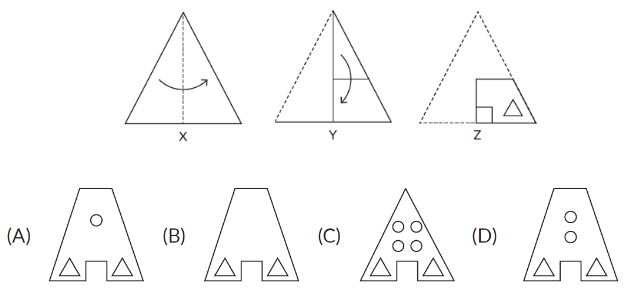(B)

Example 2: A transparent sheet of paper with markings is folded as shown in the question image. Which image would represent the sheet after the fold?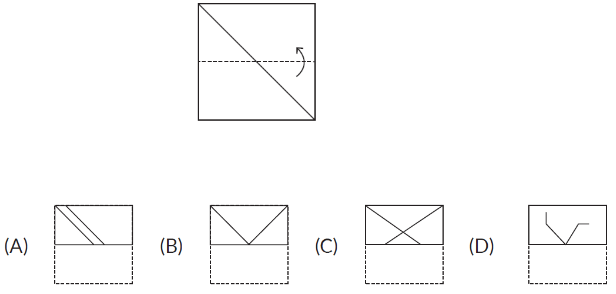(B)

## Practice Problems

Here are some questions for you to crack!!!

1. A square transparent sheet with a pattern is given. Select a figure from the options as to how the pattern would appear when the transparent sheet is folded along the dotted line.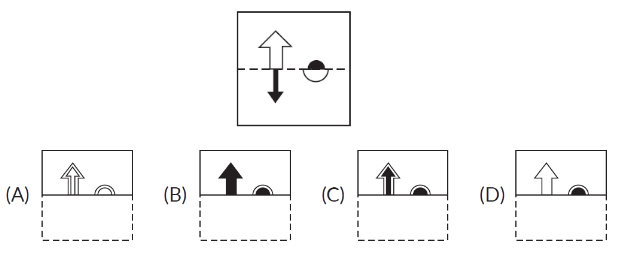2. There is a set of three figures X, Y, and Z, showing a sequence of folding of a piece of paper. Figure (Z) shows the way the folded paper has been cut. Choose the figure which would most closely resemble the unfolded form of paper.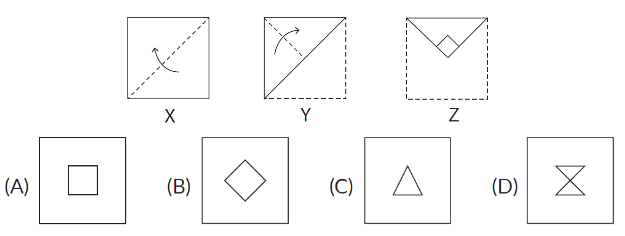Question 3: Find out which option would appear when the transparent sheet is folded at the dotted line.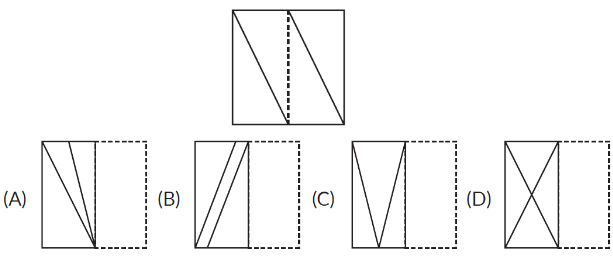4. The given question consists of a set of four figures P, Q, R, and S showing a sequence of folding of a piece of paper. Fig (S) shows the manner in which the folded paper has been cut. Select the figure from the options which would most closely resemble the unfolded form of Fig. (S).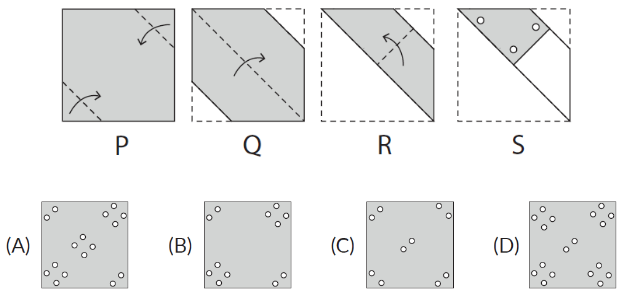1. (C)      2. (B)      3. (D)      4. (A)

## Conclusion

Hope you enjoyed this reasoning activity. These activities like paper folding and paper cutting help children to understand the concepts and fill the gap in learning them.

Learning math with these activities will aid them to scale back the evasion created with the math olympiad. Enjoy learning math!

## How to solve paper folding and cutting questions?

In Paper Folding and Cutting, a couple of figures are given describing the system in which a part is to be folded and then to be cut from a suitable section. The speckled line is the source line by which the paper is to be folded and the arrow shows the course of the overlap.

Therefore, the figures symbolize the flow during which the paper is to be folded. The patterns from the cut will appear on all of the folds formed on the paper.

-Written by Prema Jyothi, Cuemath Teacher

Related Articles
GIVE YOUR CHILD THE CUEMATH EDGE
Access Personalised Math learning through interactive worksheets, gamified concepts and grade-wise courses